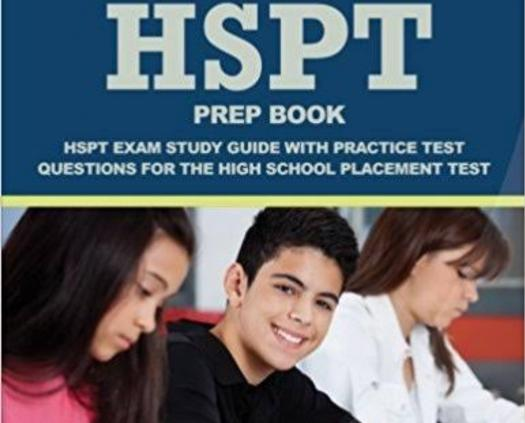# High School Placement Test Prep

Approved & Edited by ProProfs Editorial Team
The editorial team at ProProfs Quizzes consists of a select group of subject experts, trivia writers, and quiz masters who have authored over 10,000 quizzes taken by more than 100 million users. This team includes our in-house seasoned quiz moderators and subject matter experts. Our editorial experts, spread across the world, are rigorously trained using our comprehensive guidelines to ensure that you receive the highest quality quizzes.
A
Community Contributor
Quizzes Created: 948 | Total Attempts: 930,435
Questions: 10 | Attempts: 237SettingsThe Highschool placement test is a test taken by eight graders to help with admissions, scholarship selection and curriculum placement. The test is broken into 5 sections: Verbal; Quantitative; Reading; Mathematics; and Language Skills. The Highschool placement test determines whether or not the eight graders move to the ninth grader and is taken only once.

• 1.

### What angle is complementary to 55°?

• A.

23°

• B.

78°

• C.

50°

• D.

35°

D. 35°
Explanation
The angle that is complementary to another angle is the angle that, when added to the given angle, will result in a sum of 90 degrees. In this case, the angle that is complementary to 55 degrees would be 35 degrees. Adding 55 degrees and 35 degrees gives a sum of 90 degrees, which is the definition of complementary angles.

Rate this question:

• 2.

### What is the reciprocal of 20?

• A.

0.05

• B.

0.5

• C.

10

• D.

20

A. 0.05
Explanation
The reciprocal of a number is obtained by dividing 1 by the number. In this case, the reciprocal of 20 is 1/20, which is equal to 0.05.

Rate this question:

• 3.

### Why is '5' a prime number?

• A.

Its square is 25

• B.

It is only divisible by 5

• C.

It is only divisible by itself and 1

• D.

It is an odd number

C. It is only divisible by itself and 1
Explanation
The given answer states that '5' is a prime number because it is only divisible by itself and 1. This is the definition of a prime number - a number that has no other divisors except for 1 and itself. Therefore, '5' fits this criteria and is considered a prime number.

Rate this question:

• 4.

### How many seconds make a day?

• A.

36000 seconds

• B.

446900000 seconds

• C.

6000 seconds

• D.

86400 seconds

D. 86400 seconds
Explanation
There are 60 seconds in a minute, 60 minutes in an hour, and 24 hours in a day. Therefore, to calculate the total number of seconds in a day, we multiply 60 by 60 and then by 24, which equals 86,400 seconds.

Rate this question:

• 5.

### What does this statement means: "He kicked the bucket" ?

• A.

He splashed the content of the bucket

• B.

He died

• C.

He broke the plastic bucket

• D.

He lifted the bucket with his leg

B. He died
Explanation
The phrase "He kicked the bucket" is an idiom that means someone has died. It is not meant to be taken literally, but rather as a euphemism for the end of someone's life.

Rate this question:

• 6.

### When is a word considered a synonym to the other word in a sentence?

• A.

When is has exactly opposite to the highlighted word

• B.

When it has exactly the same meaning as the highlighted word

• C.

When it has the nearest meaning to the highlighted word

• D.

When it can properly replace the highlighted word

D. When it can properly replace the highlighted word
Explanation
When a word can properly replace the highlighted word in a sentence, it is considered a synonym. This means that the word has a similar meaning and can be used interchangeably without changing the overall meaning of the sentence.

Rate this question:

• 7.

### Which of this would make a good subject in a sentence?

• A.

Predicate

• B.

Noun clause

• C.

Object

• D.

Noun phrase

D. Noun phrase
Explanation
A noun phrase would make a good subject in a sentence because it consists of a noun and any words that modify or describe it. In a sentence, the subject is typically the noun phrase that performs the action or is being described. Therefore, a noun phrase is a suitable choice for a subject in a sentence.

Rate this question:

• 8.

### What is the product of the square root of 25 and the square root of 81?

• A.

400

• B.

209

• C.

345

• D.

45

D. 45
Explanation
The product of the square root of 25 and the square root of 81 can be calculated by multiplying the two square roots together. The square root of 25 is 5, and the square root of 81 is 9. Multiplying 5 and 9 gives us 45, which is the correct answer.

Rate this question:

• 9.

### Which of these describes in a sentence?

• A.

• B.

• C.

Noun phrases

• D.

Explanation
Adjectives are words that describe or modify nouns or pronouns. They provide additional information about the noun or pronoun by answering questions such as what kind, which one, how many, or how much. Adjectival phrases are groups of words that function as adjectives, providing more detailed descriptions. In this context, the correct answer is "Adjectives" because it accurately describes the category of words that provide additional information about nouns or pronouns.

Rate this question:

• 10.

### What is a synonym of the verb "stop"?

• A.

Halt

• B.

Keen

• C.

Start

• D.

Wait

A. Halt
Explanation
The synonym of the verb "stop" is "halt".

Rate this question:

Related TopicsBack to top